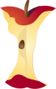Fluently Adding and Subtracting Double Digit NumbersPrintable Worksheets And Lessons

• Step-by-step Lesson- We run you through everything in this lesson. Single and double digit sums, double digit differences and sums.

• Guided Lesson - Horizontal and vertical double digit differences and sums. We follow that with a simple image based problem.

• Guided Lesson Explanation - You could also just have the students rewrite the numbers in a horizontal format, if that helps.

• Practice Worksheet - More double digit basic operations. They are all aligned vertically.

• Matching Worksheet - Match the horizontal sum of difference with its equation.(Click Here to Upgrade)

Homework Sheets

This skill, by most past state standard fell into grade 3, but now it is a grade 2 skill.

Practice Worksheets

I threw in a matching format to make it a little bit more fun for the kids.

Math Skill Quizzes

There is a good bit of number carrying going on here.

 Quiz 1 Quiz 2 Quiz 3